# Electric Dipole

### What Is an Electric Dipole?

The electric dipole is an important entity in the study of the electric field in dielectric media, which is very important for the analysis of electromagnetic optical waves in waveguides.

A system of two equal and opposite charges q separated by a small distance L is called an electric dipole as shown below.### Electric Dipole Moment

An electric dipole's strength and orientation are described by the dipole moment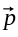, which is a vector that points from the negative charge -q toward the positive charge +q and has the magnitude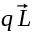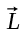is called the displacement vector pointing from the negative charge to the positive charge as shown below.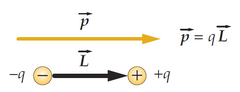The electric dipole moment is a measure of the separation of positive and negative electrical charges in a system of electric charges, that is, a measure of the charge system's overall polarity.

The SI units are Coulomb-meter (C m), however the most commonly used unit is the Debye (D).

### ExampleThe electric field on the axis of the dipole at a point a great distance |x| away is in the same direction asand has magnitude: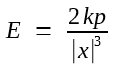At a point far from a dipole in any direction, the magnitude of the electric field is proportional to the magnitude of the dipole moment and decreases with the cube of the distance.

The equipotential lines are drawn as solid lines, and the electric field lines are drawn as dashed lines in the following figure for an electric dipole. The electric field lines are rotationally symmetrical about the z-axis (independent of azimuth angle φ) and are everywhere normal (perpendicularly) to the equipotential lines.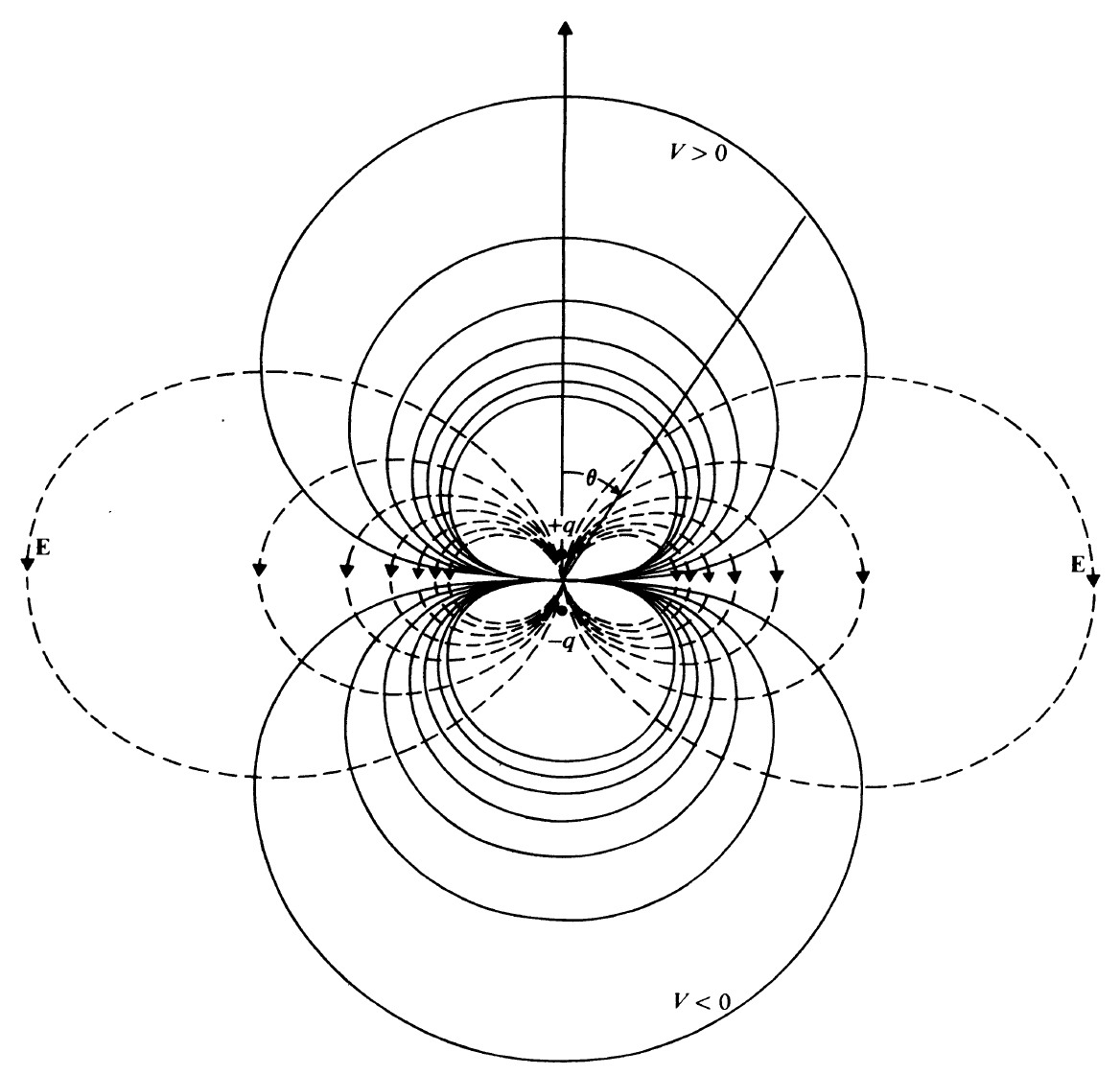Equipotential and Electric Field Lines of an Electric Dipole